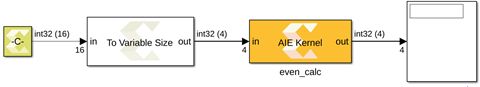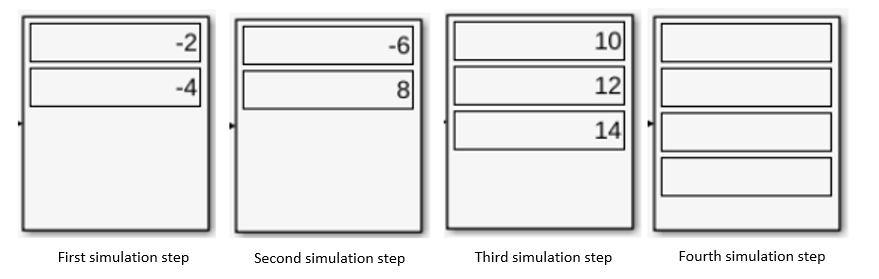# Variable-Size Signals - 2022.1 English

## Vitis Model Composer User Guide (UG1483)

Document ID
UG1483
Release Date
2022-05-26
Version
2022.1 English

In Simulink, a signal whose size (the number of elements in a dimension) can change during Simulink simulation is called a variable-size signal. To understand the importance of variable-size signaling in the context of modeling an AI Engine design in Model Composer, consider a kernel that outputs even numbers from a set of input numbers.

``````void even_calc(input_window_int32 * in, output_stream_int32 * out) {
int32 val,temp;
for (unsigned i=0; i<4; i++) {
if(val % 2 == 0) {
int32 temp = val;
writeincr(out,temp);
}
}
}``````

Here, the input is a window of type `int32` and the output is a stream of similar type. If you try to model this in Simulink, you will observe that the number of data samples it produces may vary at each invocation.

Assume the input window size is `4` and the input given to the kernel is ```[1 -2 3 -4 5 -6 7 8 9 10 12 14 5 7 9 13]```.

Figure 1. Variable-Size SignalsTo understand why the size of the output can vary dynamically, run the simulations in steps.

During the first simulation step, the AI Engine kernel consumes four values (i.e., `1,-2,3,-4`) and outputs two values (`[-2,-4]`), which are the even numbers in the set [`1,-2,3,-4`]. In the second simulation step, the kernel consumes the next set of window inputs (i.e, `[5,-6,7 ,8]`) the output is [`-6,8`]. For the third set of inputs [`9,10,12,14`] , the output at the third simulation step is [`10,12,14`]. Similarly for the final set of inputs [`5,7,9,13`], the output is empty because there are no even numbers to produce from the fourth input window.

In summary, the output size at the:

• First simulation step is `2`.
• Second simulation step is `2`.
• Third simulation step is `3`.
• Fourth simulation step is `0`.
Figure 2. Display Block - Simulation StepsSo, the kernel produces data samples of different sizes at every invocation and sometimes it does not produce any output. To model this behavior in Model Composer, you can use variable-size signals in Simulink. However, during a simulation, the number of dimensions cannot change. A variable-size signal always has an associated maximum signal size. In this case, it is `4`.

The variable-size signal in Simulink is represented with a thicker signal line unlike the normal signal as highlighted in the previous figure. You can learn more about variable size signals at the following links: